# R语言randomForest包的随机森林分类模型以及对重要变量的选择

08/24 17:01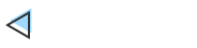R包randomForest的随机森林分类模型以及对重要变量的选择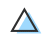1）假设训练集中共有N个对象、M个变量，从训练集中随机有放回地抽取N个对象构建决策树；

2）在每一个节点随机抽取m<M个变量，将其作为分割该节点的候选变量，每一个节点处的变量数应一致；

3）完整生成所有决策树，无需剪枝（最小节点为1）；

4）重复（1-3）过程，获得大量决策树；终端节点的所属类别由节点对应的众数类别决定；

5）对于新的观测点，用所有的树对其进行分类，其类别由多数决定原则生成。

https://pan.baidu.com/s/10MWBfjBnYIzf6Cx2Zd9CjA

1）任一OTUs的丰度都很难作为判别两种不同环境的标准，因此接下来综合考虑所有OTUs的丰度并进行建模，目的是找到能够稳定区分两种环境的代表性OTUs组合（作为生物标志物）；

2）通过代表性OTUs的丰度构建预测模型，即仅通过这些OTUs的丰度就能够判断样本分类。

#读取 OTUs 丰度表otu <- read.table('otu_table.txt', sep = '\t', row.names = 1, header = TRUE, fill = TRUE) #过滤低丰度 OTUs 类群，它们对分类贡献度低，且影响计算效率#120 个样本，就按 OTUs 丰度的行和不小于 120 为准吧otu <- otu[which(rowSums(otu) >= 120), ] #合并分组，得到能够被 randomForest 识别计算的格式group <- read.table('group.txt', sep = '\t', row.names = 1, header = TRUE, fill = TRUE)otu <- data.frame(t(otu))otu_group <- cbind(otu, group) #将总数据集分为训练集（占 70%）和测试集（占 30%）set.seed(123)select_train <- sample(120, 120*0.7)otu_train <- otu_group[select_train, ]otu_test <- otu_group[-select_train, ]

randomForest包方法的细节介绍可参考：

https://www.stat.berkeley.edu/~breiman/RandomForests/

#randomForest 包的随机森林library(randomForest) #随机森林计算（默认生成 500 棵决策树），详情 ?randomForestset.seed(123)otu_train.forest <- randomForest(groups ~ ., data = otu_train, importance = TRUE)otu_train.forest plot(margin(otu_train.forest, otu_train$groups), main = '观测值被判断正确的概率图')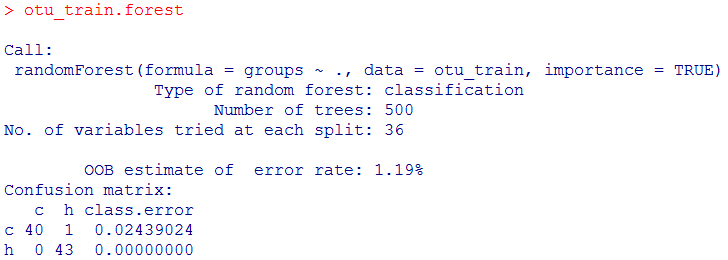randomForest()函数从训练集中有放回地随机抽取84个观测点，在每棵树的每个节点随机抽取36个变量，从而生成了500棵经典决策树。 生成树时没有用到的样本点所对应的类别可由生成的树估计，与其真实类别比较即可得到袋外预测（out-of-bagOOB）误差，即OOB estimate of error rate，可用于反映分类器的错误率。此处为为1.19%，显示分类器模型的精准度是很高的，可以有效识别两类分组。 Confusion matrix比较了预测分类与真实分类的情况，class.error代表了错误分类的样本比例，这里是很低的：组的41个样本中40个正确分类，h组的43个样本全部正确分类。概率图显示绝大部分样本的分类具有非常高的正确率。 若识别模糊，则会出现偏离。 分类器性能测试 不妨使用构建好的分类器分类训练集样本，查看判别的样本分类情况。 #训练集自身测试train_predict <- predict(otu_train.forest, otu_train)compare_train <- table(train_predict, otu_train$groups)compare_trainsum(diag(compare_train)/sum(compare_train))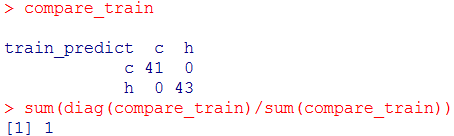#使用测试集评估test_predict <- predict(otu_train.forest, otu_test)compare_test <- table(otu_test$groups, test_predict, dnn = c('Actual', 'Predicted'))compare_test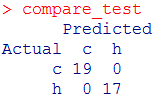显示初步构建的随机森林分类器能够准确分类训练集外的样本。 寻找代表性的OTUs组合 变量重要性 随机森林除了分类器外的另一常用功能是识别重要的变量，即计算变量的相对重要程度。 在这里，就是期望寻找能够稳定区分两种环境的代表性OTUs组合（作为生物标志物）。 ##关键 OTUs 识别#查看表示每个变量（OTUs）重要性的得分#summary(otu_train.forest)importance_otu <- otu_train.forest$importancehead(importance_otu) #或者使用函数 importance()importance_otu <- data.frame(importance(otu_train.forest))head(importance_otu) #可以根据某种重要性的高低排个序，例如根据“Mean Decrease Accuracy”指标importance_otu <- importance_otu[order(importance_otu$MeanDecreaseAccuracy, decreasing = TRUE), ]head(importance_otu) #输出表格#write.table(importance_otu, 'importance_otu.txt', sep = '\t', col.names = NA, quote = FALSE)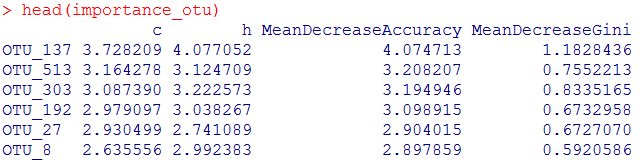此处“Mean Decrease Accuracy”和“Mean Decrease Gini”为随机森林模型中的两个重要指标。其中，“mean decrease accuracy”表示随机森林预测准确性的降低程度，该值越大表示该变量的重要性越大；“mean decrease gini”计算每个变量对分类树每个节点上观测值的异质性的影响，从而比较变量的重要性。该值越大表示该变量的重要性越大。 到这一步，可从中筛选一些关键OTUs作为代表物种，作为有效区分两种环境的生物标志物。 该图展示了其中top30关键的OTUs，将它们划分为“关键OTUs”的依据为模型中的两个重要指标（两个指标下各自包含30OTUs，默认由高往低排）。 #作图展示 top30 重要的 OTUsvarImpPlot(otu_train.forest, n.var = min(30, nrow(otu_train.forest$importance)), main = 'Top 30 - variable importance')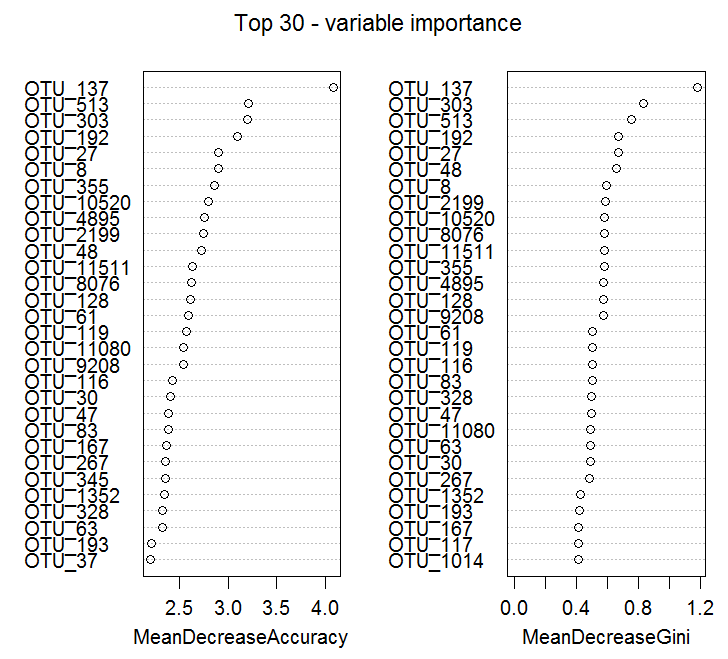##交叉验证帮助选择特定数量的 OTUs#5 次重复十折交叉验证set.seed(123)otu_train.cv <- replicate(5, rfcv(otu_train[-ncol(otu_train)], otu_train$group, cv.fold = 10,step = 1.5), simplify = FALSE)otu_train.cv #提取验证结果绘图otu_train.cv <- data.frame(sapply(otu_train.cv, '[[', 'error.cv'))otu_train.cv$otus <- rownames(otu_train.cv)otu_train.cv <- reshape2::melt(otu_train.cv, id = 'otus')otu_train.cv$otus <- as.numeric(as.character(otu_train.cv$otus)) #拟合线图library(ggplot2)library(splines)  #用于在 geom_smooth() 中添加拟合线，或者使用 geom_line() 替代 geom_smooth() 绘制普通折线 p <- ggplot(otu_train.cv, aes(otus, value)) +    geom_smooth(se = FALSE,  method = 'glm', formula = y~ns(x, 6)) +    theme(panel.grid = element_blank(), panel.background = element_rect(color = 'black', fill = 'transparent')) +    labs(title = '',x = 'Number of OTUs', y = 'Cross-validation error') p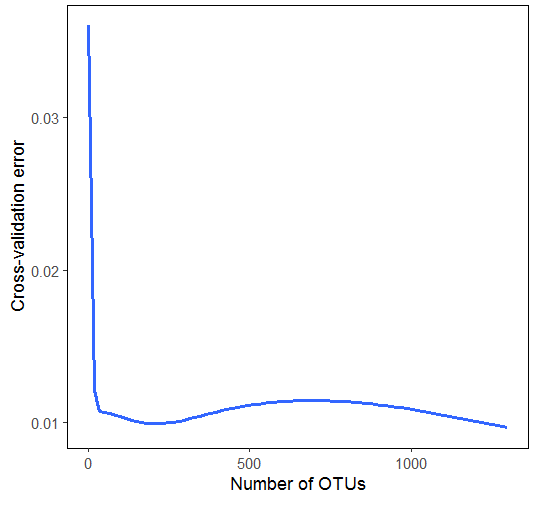#大约提取前 30 个重要的 OTUsp + geom_vline(xintercept = 30) #根据 OTUs 重要性排序后选择，例如根据“Mean Decrease Accuracy”指标importance_otu <- importance_otu[order(importance_otu$MeanDecreaseAccuracy, decreasing = TRUE), ]head(importance_otu) #输出表格#write.table(importance_otu[1:30, ], 'importance_otu_top30.txt', sep = '\t', col.names = NA, quote = FALSE)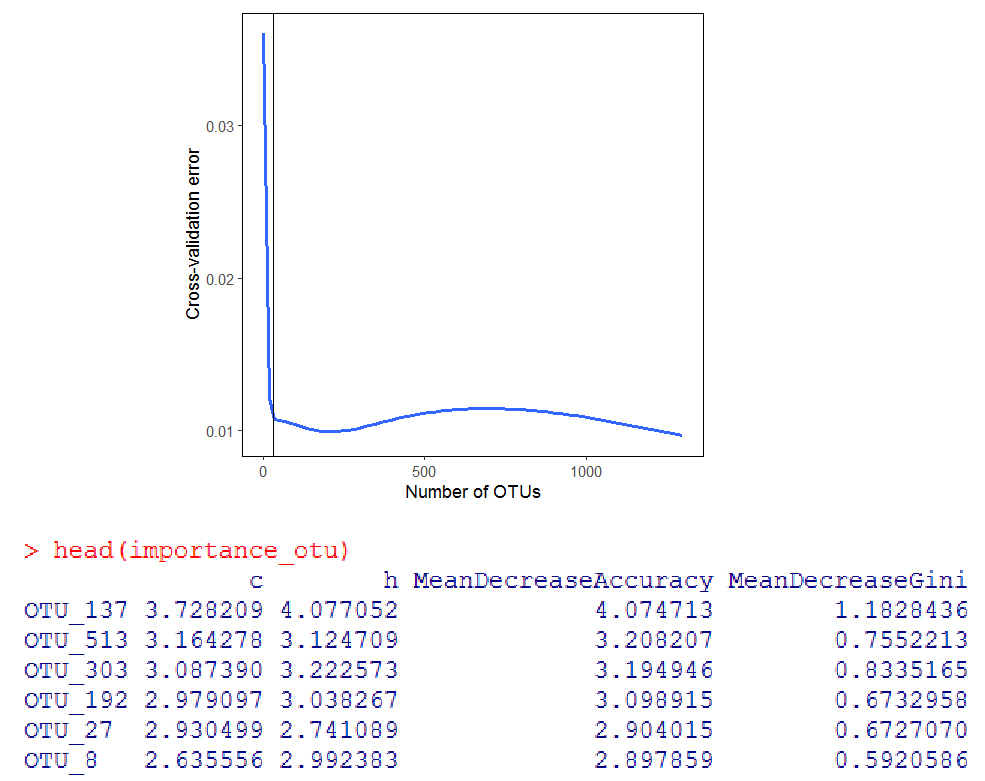简约模型 如上的交叉验证曲线可反映出，并非所有变量都有助于预测模型，重要性排名靠后的变量的效应不明显甚至存在着负效应。就本文的示例而言，有些OTUs对于分类的贡献度并不高，有些可能在组间区别不大甚至会增加错误率。 因此，对于一开始构建的随机森林分类器，很多变量其实是可以剔除的。不妨就以上述选择的前30个最重要的OTUs代替原数据集中所有的OTUs进行建模，一方面助于简化分类器模型，另一方面还可提升分类精度。 ##简约分类器#选择 top30 重要的 OTUs，例如上述已经根据“Mean Decrease Accuracy”排名获得otu_select <- rownames(importance_otu)[1:30] #数据子集的训练集和测试集otu_train_top30 <- otu_train[ ,c(otu_select, 'groups')]otu_test_top30 <- otu_test[ ,c(otu_select, 'groups')] #随机森林计算（默认生成 500 棵决策树），详情 ?randomForestset.seed(123)otu_train.forest_30 <- randomForest(groups ~ ., data = otu_train_top30, importance = TRUE)otu_train.forest_30 plot(margin(otu_train.forest_30, otu_test_top30$groups), main = '观测值被判断正确的概率图') #训练集自身测试train_predict <- predict(otu_train.forest_30, otu_train_top30)compare_train <- table(train_predict, otu_train_top30$groups)compare_train #使用测试集评估test_predict <- predict(otu_train.forest_30, otu_test_top30)compare_test <- table(otu_test_top30$groups, test_predict, dnn = c('Actual', 'Predicted'))compare_test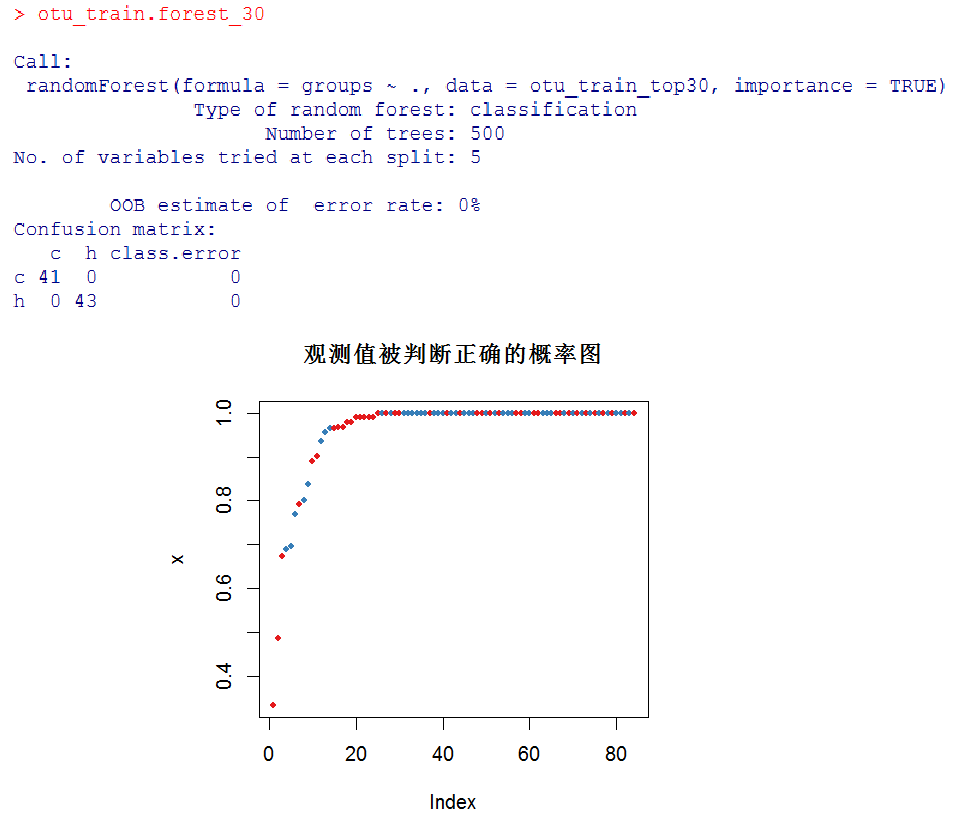#训练集自身测试train_predict <- predict(otu_train.forest_30, otu_train_top30)compare_train <- table(train_predict, otu_train_top30$groups)compare_train #使用测试集评估test_predict <- predict(otu_train.forest_30, otu_test_top30)compare_test <- table(otu_test_top30$groups, test_predict, dnn = c('Actual', 'Predicted'))compare_test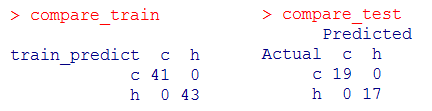##NMDS 排序图中展示分类#NMDS 降维nmds <- vegan::metaMDS(otu, distance = 'bray')result <- nmds$pointsresult <- as.data.frame(cbind(result, rownames(result))) #获得上述的分类预测结果predict_group <- c(train_predict, test_predict)predict_group <- as.character(predict_group[rownames(result)]) #作图colnames(result)[1:3] <- c('NMDS1', 'NMDS2', 'samples')result$NMDS1 <- as.numeric(as.character(result$NMDS1))result$NMDS2 <- as.numeric(as.character(result$NMDS2))result$samples <- as.character(result\$samples)result <- cbind(result, predict_group)head(result) ggplot(result, aes(NMDS1, NMDS2, color = predict_group)) +    geom_polygon(data = plyr::ddply(result, 'predict_group', function(df) df[chull(df[], df[]), ]), fill = NA) +    geom_point()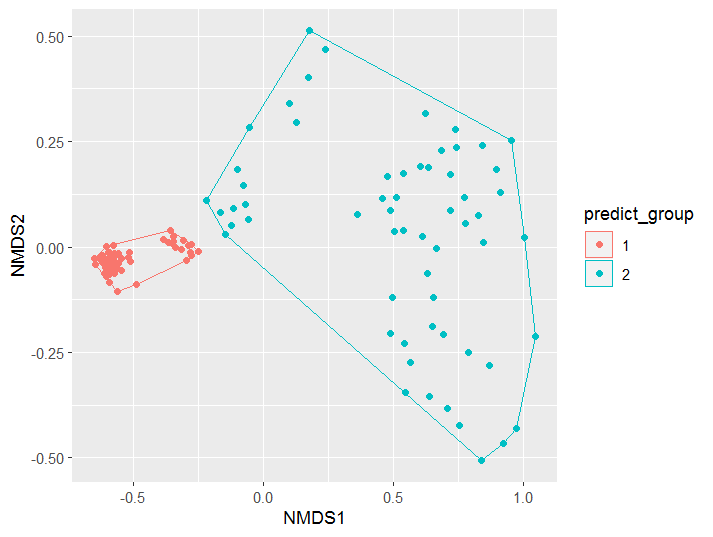R包ropls的偏最小二乘判别分析（PLS-DA）和正交偏最小二乘判别分析（OPLS-DA）

R包tidyLPA的潜剖面分析（LPA）

R包poLCA的潜类别分析（LCA）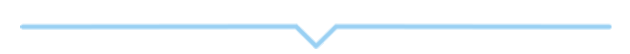0
0 收藏

### 作者的其它热门文章0 评论
0 收藏
0